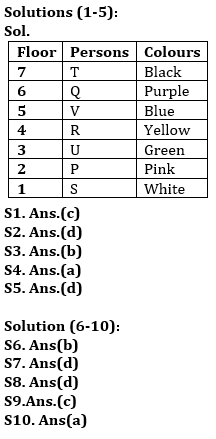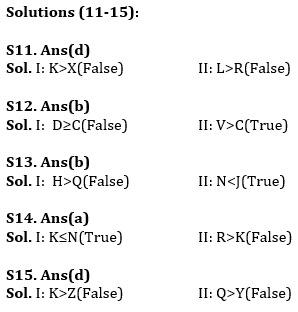Latest Banking jobs   »   Reasoning Ability Quiz For IBPS RRB...

# Reasoning Ability Quiz For IBPS RRB PO, Clerk Prelims 2021- 27th July

Directions (1-5): Read the following information carefully and answer the given questions:
Seven persons are living in a different floor of a building having seven floors, lowermost floor is numbered as 1 and topmost floor is numbered as 7. They like different colours- Blue, Pink, Black, Purple, Green, Yellow, and White (but not necessarily in the same order). P likes Pink and lives on an even numbered floor but not more than two people live below P. V lives on 5th floor and does not like Yellow. T likes Black and lives on one of the odd numbered floors above the one likes Blue. Q likes Purple. R lives just above U, who likes Green. S likes White and lives below P.

Q1. Who among the following likes Blue?
(a) Q
(b) P
(c) V
(d) S
(e) None of these

Q2. Who among the following lives just below the one who likes Yellow?
(a) U
(b) S
(c) The one who likes Green
(d) Both (a) and (c)
(e) None of these

Q3. R lives on which of the following floor?
(a) 3rd
(b) 4th
(c) 5th
(d) 7th
(e) None of these

Q4. How many persons live between Q and P?
(a) Three
(b) Two
(c) One
(d) Four
(e) None of these

Q5. Who among the following lives just above V?
(a) T
(b) S
(c) P
(d) Q
(e) None of these

Directions (6-10): Study the following alphanumeric series carefully and answer the questions given below:

7 6 @ 5 R H 6 1 2 8 # E D 6 6 5 2 3 * M K A % U \$ 2 F 9 \$ T 3 1 H J

Q6. Which of the following element is 21th from right end of the series?
(a) D
(b) 6
(c) 5
(d) 2
(e) None of these

Q7. How many such symbols are there which is preceded by numbers and followed by vowels?
(a) Four
(b) None
(c) Two
(d) One
(e) Three

Q8. How many such numbers are there which is preceded by alphabets and followed by symbols?
(a) Four
(b) Two
(c) Three
(d) One
(e) None

Q9. If all numbers are eliminated from the series then, which of the following element is 9th from left end of the series?
(a) *
(b) M
(c) K
(d) A
(e) %

Q10.How many alphabets are there between the 9th element from left end and 14th element from right end?
(a) Three
(b) Two
(c) One
(d) Four
(e) More than three

Directions (11-15): In these questions, relationship between different elements is show in the statements. The statements are followed by conclusions. Study the conclusions based on the given statements and select the appropriate answer:
(a) If only conclusion I follows.
(b) If only conclusion II follows.
(c) If either conclusion I or II follows
(d) If neither conclusion I nor II follows.
(e) If both conclusions I and II follow.

Q11. Statements: V>J>X<L=F≥R<U<Q≤K
Conclusion
I: K>X
II: L>R

Q12. Statements: D>R=L>C<N≤V>F<L
Conclusion
I: D≥C
II: V>C

Q13. Statements: H≥B=Q>I<J>S>N≤L
Conclusion
I: H>Q
II: N<J

Q14. Statements: C<N=F≥K>E<T≤R≥Y
Conclusion
I: K≤N
II: R>K

Q15. Statements: Y>Z>U>Q≤K≥L=Q
Conclusion
I: K>Z
II: Q>Y

Practice More Questions of Reasoning for Competitive Exams:

###### Study Plan for IBPS RRB PO/Clerk Prelims 2021

Solutions